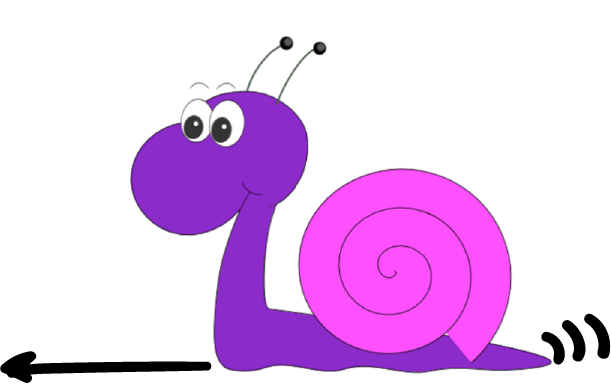# Solution: Qualifying for the Snail Olympics Puzzle

## The Puzzle:Gertie the snail is at the trials for the Snail Olympics. The qualifying speed for the olympics is $24 \text{ cm/h}$, and she has completed one lap of the course at a speed of $12 \text{ cm/h}$. How fast does she need to go on the second lap to make her average speed for the two lap trial equal the qualifying speed?

## The Solution:

Unfortunately, Gertie has missed out this year. Maybe she'll make it for the next Olympiad.

Let's do some algebra to see why.

Let $d$ cm be the length of one lap of the course, let $t$ be the time (in hours) taken to complete a lap at $12 \text{ cm/h}$, and let $t'$ be the time (in hours) required to complete one lap of the course at this new, faster speed.

Then Gertie needs to travel a total distance of $2d \text{ cm}$ to complete two laps of the course, and she will do this in $t + t'$ hours. Since $\text{speed} = \dfrac{\text{distance travelled}}{\text{time taken}}$, we require $24 = \dfrac{2d}{t + t'}$, so $24 t + 24t' = 2d$.

But, she completes one lap of the course at $12 \text{ cm/h}$ in $t$ hours. So, $d = 12t$ and $2d = 24 t$. Plugging this into the equation gives

$\begin{eqnarray*} 24t + 24t' &= 24t\\ 24t' &= 0\\ t' &= 0 \end{eqnarray*}$
So, Gertie would have to complete the second lap in no time at all! In other words, her speed would have to be infinite.

For example, let's suppose that the course is $12 \text{ cm}$ long. Gertie completes the first lap of the course in one hour at $12 \text{ cm/h}$. The total length of two laps is $24 \text{ cm}$, so she would have to complete the two lap trial in $1 \text{ hour}$ to have an average speed of $24 \text{ cm/h}$. This time has already elapsed, and Gertie is only halfway through. So, there's no way that Gertie can possibly reach the qualifying speed for the Olympics. Better luck next time, Gertie!

### Description

This series of puzzles are for Year 10 or higher students, these puzzles tests your skills and also train you with problem solving and thinking out of the box

### Audience

Year 10 students or higher

### Learning Objectives

Solving puzzles

Author: Subject Coach
You must be logged in as Student to ask a Question.